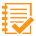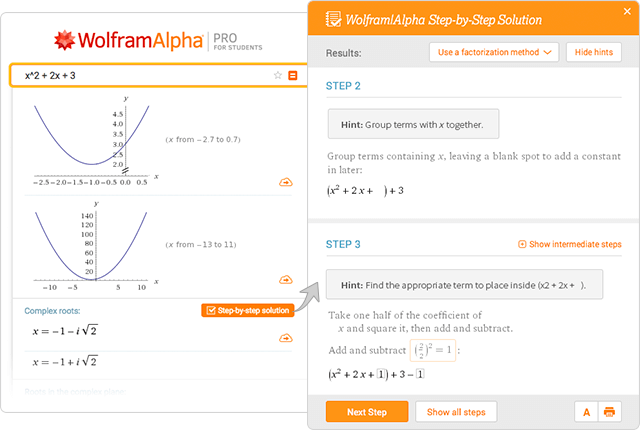Pro

Examples for

#Step-by-Step Solutions

Solutions for over 200 topics within math, chemistry and physics problems across all levels of education, from elementary school to college.Arithmetic

More examples
Statistics

#### Perform basic statistical analysis on a set of data, seeing the steps along the way:

More examples
Discrete Mathematics

More examples
Chemistry

More examples
Algebra

More examples
Calculus

More examples
Linear Algebra

More examples
Physics

More examples
Geometry

#### Determine the equation of a line with certain properties using a selected form:

More examples
Differential Equations

More examples
Proofs

More examples